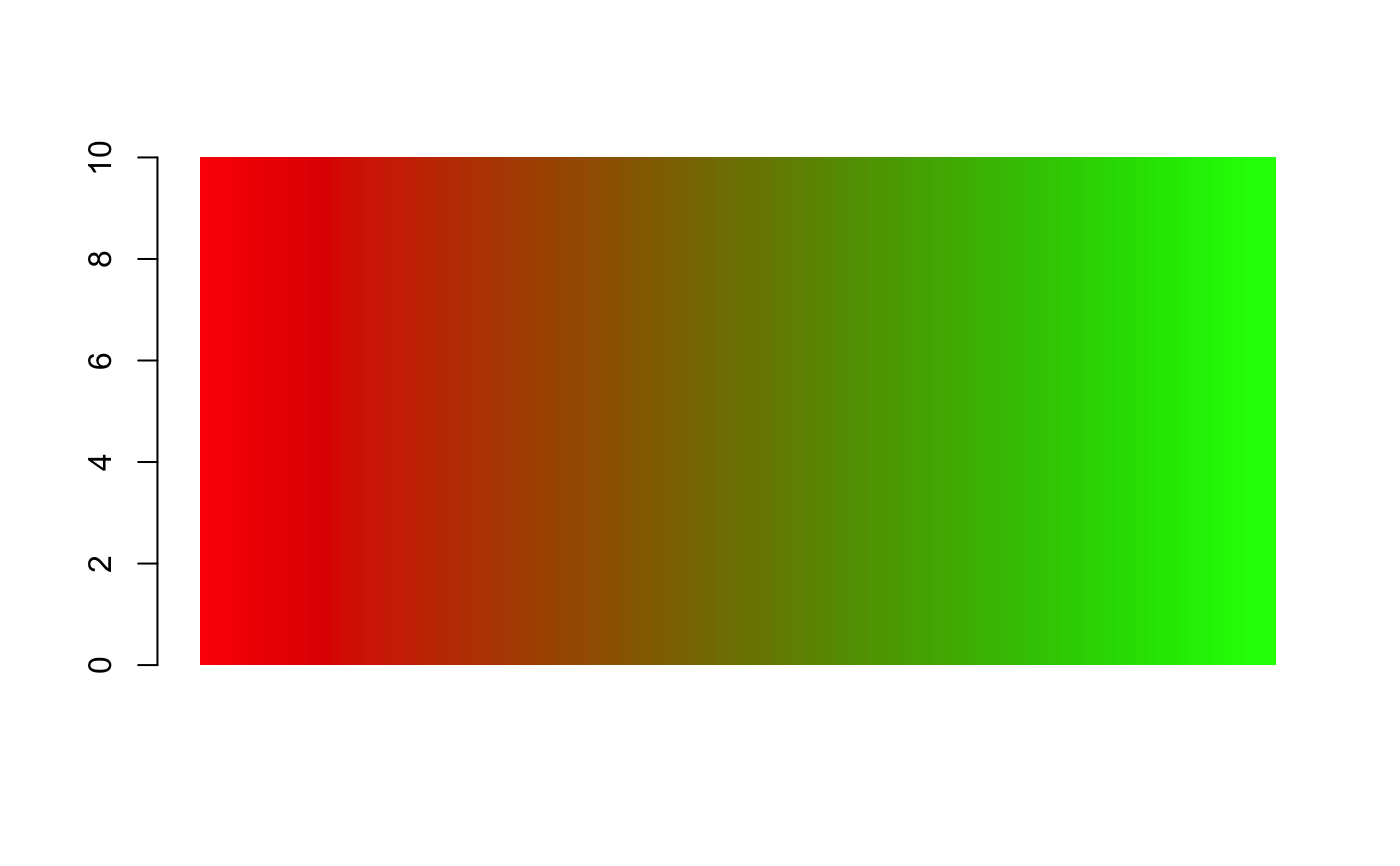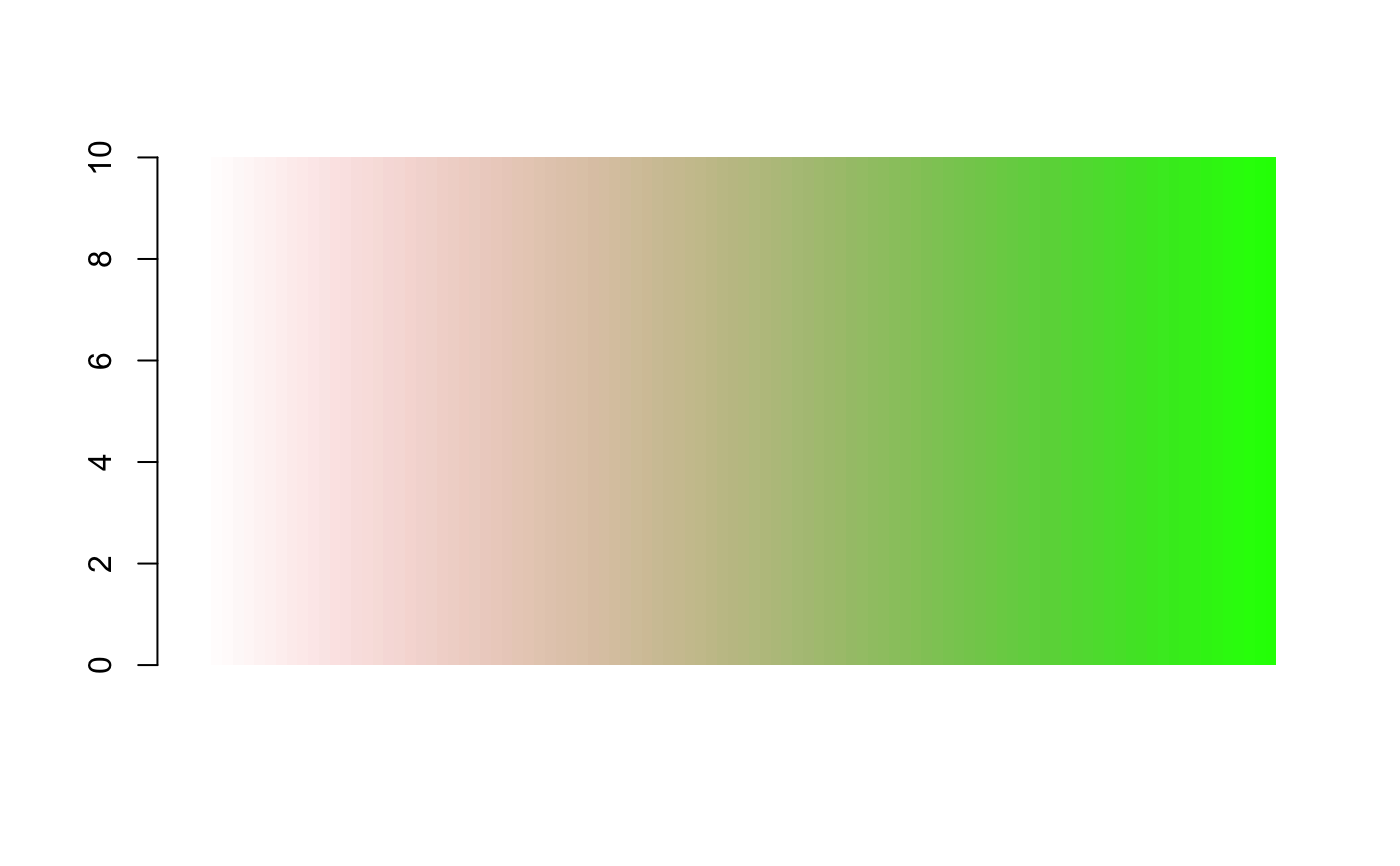maps colours to values

colour_values(x, palette = "viridis", na_colour = "#808080FF",
alpha = 255, include_alpha = TRUE, ...)

color_values(x, palette = "viridis", na_colour = "#808080FF",
alpha = 255, include_alpha = TRUE, ...)

# S3 method for character
colour_values_to_hex(x, palette, na_colour, alpha,
include_alpha, summary = FALSE)

# S3 method for default
colour_values_to_hex(x, palette, na_colour, alpha,
include_alpha, n_summaries = 0, format = TRUE, digits = 2)

# S3 method for logical
colour_values_to_hex(x, palette, na_colour, alpha,
include_alpha, summary = FALSE)

# S3 method for factor
colour_values_to_hex(x, palette, na_colour, alpha,
include_alpha, summary = FALSE)

# S3 method for Date
colour_values_to_hex(x, palette, na_colour, alpha,
include_alpha, n_summaries = 0, format = TRUE)

# S3 method for POSIXct
colour_values_to_hex(x, palette, na_colour, alpha,
include_alpha, n_summaries = 0, format = TRUE)

# S3 method for POSIXlt
colour_values_to_hex(x, palette, na_colour, alpha,
include_alpha, n_summaries = 0, format = TRUE)

## Arguments

x vector of values to map to a colour colour palette. See details and examples hex string colour to use for NA values in the form #RRGGBBAA. optional. Single value in [0,255] applied to all colours, or a decimal in [0, 1) (to indicate a percentage, noting 1 is excluded), or a vector of numeric values the same length as x. The numeric vector will be scaled into the range [0,255]. If a matrix palette is supplied this argument is ignored. logical indicating if the returned hex or matrix should include the alpha values. Defaults to TRUE. other arguments possed to methods logical indicating if a summary of the colours should be returned as well as the full colour mapping. This will be the unique elements of x mapped to the colour. positive integer. If supplied a summary colour palette will be returned in a list, containing n_summaries equally spaced values of x in the range [min(x),max(x)], and their associated colours. If a non-numeric x is used this value is ignored logical indicating if the summary values should be formatted. See details Integer. When summarising a numeric vector you can specify the number of decimal places to include in the summary values

## Details

The palette can either be

• String - use colour_palettes() to view available palettes

• Matrix - At least 5 rows, and 3 (or 4) columns representing the red, green and blue (and alpha) values

The matrix palette requires 5 rows because the colours are interpolated using a cubic b-spline. This method requires 5 values.

when summary = TRUE, the following rules are applied to the summary values

• logical vectors are converted to "TRUE" or "FALSE" strings

• all other types remain as-is, unless format = T is used

when format = TRUE,

• numbers are converted to strings with the specified number of decimal places (using digits argument)

• Dates are formatted as "yyyy-mm-dd"

## See also

colour_values_rgb

## Examples


## in-built palettes
colour_values(x = 1:5) ## default is "viridis"#>  "#440154FF" "#3B528BFF" "#21908CFF" "#5DC963FF" "#FDE725FF"colour_values(x = 1:5, palette = "inferno")#>  "#000004FF" "#57106DFF" "#BB3755FF" "#F98D0AFF" "#FCFFA4FF"colour_values(x = 1:5, palette = "plasma")#>  "#0D0887FF" "#7E03A8FF" "#CB4778FF" "#F89441FF" "#F0F921FF"colour_values(x = 1:5, palette = "magma")#>  "#000004FF" "#50127CFF" "#B63779FF" "#FB8761FF" "#FCFDBFFF"colour_values(x = 1:5, palette = "cividis")#>  "#00204DFF" "#414D6BFF" "#7C7B78FF" "#BDAF6FFF" "#FFEA46FF"colour_values(x = 1:5, palette = "rainbow")#>  "#FF0000FF" "#81FF00FF" "#00FFFCFF" "#7C00FFFF" "#FF0006FF"
## matrix palette
n <- 100
m <- grDevices::colorRamp(c("red", "green"))( (1:n)/n )
df <- data.frame(a = 10, x = 1:n)
df$col <- colour_values(df$x, palette = m)
barplot(height = df$a, col = df$col, border = NA, space = 0)## with an alpha column on the palette
n <- 100
m <- grDevices::colorRamp(c("red", "green"))( (1:n)/n )
m <- cbind(m, seq(0, 255, length.out = 100))
df <- data.frame(a = 10, x = 1:n)
df$col <- colour_values(df$x, palette = m)
barplot(height = df$a, col = df$col, border = NA, space = 0)## single alpha value for all colours
df <- data.frame(a = 10, x = 1:255)
df$col <- colour_values(df$x, alpha = 50)
barplot(height = df$a, col = df$col, border = NA, space = 0)## vector of alpha values
df <- data.frame(a = 10, x = 1:300, y = rep(c(1:50, 50:1), 3) )
df$col <- colour_values(df$x, alpha = df$y) barplot(height = df$a, col = df$col, border = NA, space = 0)## returning a summary palette colour_values(-10:10, n_summaries = 5)#>$colours
#>   "#440154FF" "#481466FF" "#482575FF" "#463480FF" "#414487FF" "#3B528BFF"
#>   "#345F8DFF" "#2F6C8EFF" "#2A788EFF" "#25848EFF" "#21908CFF" "#1E9C89FF"
#>  "#22A884FF" "#2FB47CFF" "#43BF71FF" "#5DC963FF" "#7AD151FF" "#9AD93DFF"
#>  "#BCDF27FF" "#DDE318FF" "#FDE725FF"
#>
#> $summary_values #>  "-10.00" "-5.00" "0.00" "5.00" "10.00" #> #>$summary_colours
#>  "#440154FF" "#3B528BFF" "#21908CFF" "#5DC963FF" "#FDE725FF"
#>